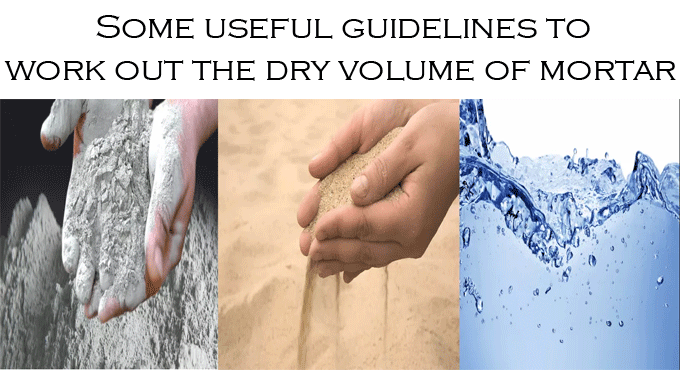# Some useful guidelines to work out the dry volume of mortarThe dry volume of mortar is measured for determining the quantity of cement and sand for the formation of mortar for our masonry work.

In recent times, cement mortar is frequently utilized for different types of masonry work.

While making estimate of the volume of mortar for various types of masonry works, only the wet volume is obtained. In order to find out the necessary volume for cement and sand, the wet volume of mortar should be changed into the dry volume.

The Dry Volume of Mortar
The prime components of mortar are cement and sand.

The mortar is formed with the inclusion of water to the dry mix of cement-sand.

After adding the water to the dry mix of cement-sand, the volume of dry mix is decreased because of the existence of air voids in sand particles.

Since the volume of mortar is raised in dry conditions, it is required to multiply the wet volume with 1.30 to obtain the dry volume of mortar.

How to work out the volume of cement and sand in mortar.

Suppose, 10 cubic feet wet mortar is required and the proportion of cement and sand in the mortar is 1:4.

Given below, the detail process to work out the dry volume mortar and then sand and cement quantity for this 10 cubic feet mortar.

How to work out the dry volume of mortar
It is known that the dry volume of mortar is,
= Wet volume of mortar x 1.30
=10 x 1.30
=13.00 cubic feet.

The process for measuring cement and sand volume in mortar

The proportion of cement and sand in mortar is 1:4. It means one unit of cement will be blended with four units of sand.

Total units of elements in mortar are 1+4=5.
Therefore, the necessary volume of sand for the projected mortar quantity is,
= (13.00 ? 5) ? 4
= 10.40 cubic feet.

Categories of chains applied in surveying:

And necessary cement quantity is,
= (13.00 ?5) x 1
= 2.60 cubic feet.

Conclusion: To measure the dry volume of mortar, it is required to calculate the wet volume of mortar for your work item and then multiply that wet volume by 1.30.

As soon as you obtain the required dry volume of mortar, then it is possible to compute the required cement and sand quantity for the mortar on the basis of the mix proportion.